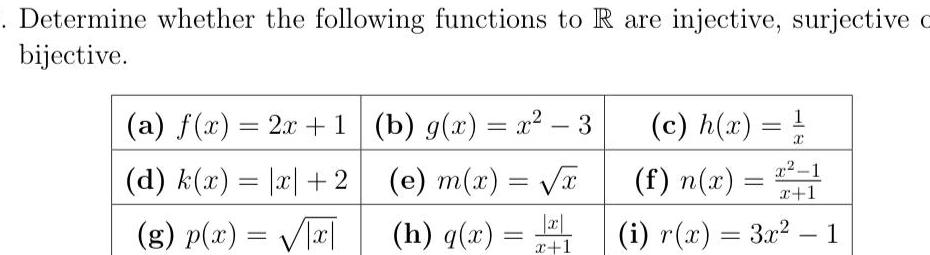Question:

# Determine whether the following functions to R are injective

Last updated: 11/16/2023Determine whether the following functions to R are injective surjective c bijective a f x 2x 1 d k x x 2 g p x x b g x x 3 e m x x h q x x x 1 1 c h x x 1 x 1 i r x 3x 1 f n x